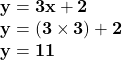## Which of the following is NOT a solution to the linear equation y=3x+2? Select the correct answer below: (1,5) (2,8)

Question

Which of the following is NOT a solution to the linear equation y=3x+2?
(1,5)
(2,8)
(3,10)
(4,14)

in progress 0
1 month 2021-08-15T05:26:07+00:00 2 Answers 1 views 0

1. (3,10) because if you were to plug in each, you would see they all work expect for (3,10) which gives you 10= 9+2